# bwboundaries

Trace region boundaries in binary image

## Syntax

``B = bwboundaries(BW)``
``B = bwboundaries(BW,conn)``
``B = bwboundaries(BW,conn,options)``
``[B,L] = bwboundaries(___)``
``````[B,L,n,A] = bwboundaries(___)``````

## Description

example

````B = bwboundaries(BW)` traces the exterior boundaries of objects, as well as boundaries of holes inside these objects, in the binary image `BW`. `bwboundaries` also descends into the outermost objects (parents) and traces their children (objects completely enclosed by the parents). The function returns `B`, a cell array of boundary pixel locations.```
````B = bwboundaries(BW,conn)` traces the exterior boundaries of objects, where `conn` specifies the connectivity to use when tracing parent and child boundaries.```

example

````B = bwboundaries(BW,conn,options)` traces the exterior boundaries of objects, where `options` is either `"holes"` or `"noholes"`, specifying whether you want to include the boundaries of holes inside other objects.```

example

````[B,L] = bwboundaries(___)` also returns a label matrix `L` where objects and holes are labeled. ```

example

``````[B,L,n,A] = bwboundaries(___)``` also returns `n`, the number of objects found, and `A`, an adjacency matrix.```

## Examples

collapse all

Read grayscale image into the workspace.

`I = imread('rice.png');`

Convert grayscale image to binary image using local adaptive thresholding.

`BW = imbinarize(I);`

Calculate boundaries of regions in image and overlay the boundaries on the image.

```[B,L] = bwboundaries(BW,'noholes'); imshow(label2rgb(L, @jet, [.5 .5 .5])) hold on for k = 1:length(B) boundary = B{k}; plot(boundary(:,2), boundary(:,1), 'w', 'LineWidth', 2) end```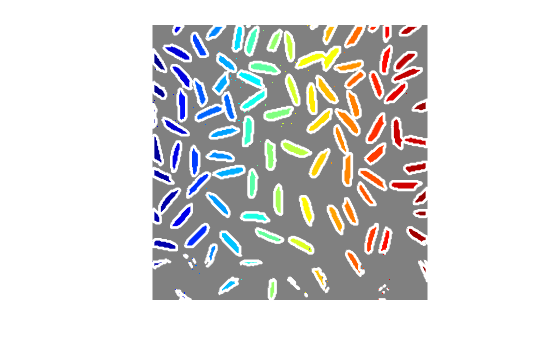Read binary image into the workspace.

`BW = imread('blobs.png');`

Calculate boundaries of regions in the image.

`[B,L,N,A] = bwboundaries(BW);`

Display the image with the boundaries overlaid. Add the region number next to every boundary (based on the label matrix). Use the zoom tool to read individual labels.

```imshow(BW); hold on; colors=['b' 'g' 'r' 'c' 'm' 'y']; for k=1:length(B), boundary = B{k}; cidx = mod(k,length(colors))+1; plot(boundary(:,2), boundary(:,1),... colors(cidx),'LineWidth',2); %randomize text position for better visibility rndRow = ceil(length(boundary)/(mod(rand*k,7)+1)); col = boundary(rndRow,2); row = boundary(rndRow,1); h = text(col+1, row-1, num2str(L(row,col))); set(h,'Color',colors(cidx),'FontSize',14,'FontWeight','bold'); end```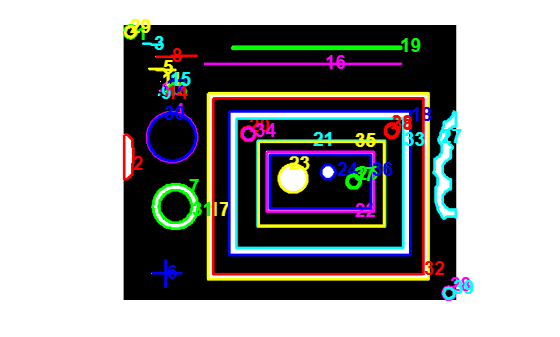Display the adjacency matrix using the `spy` function.

```figure spy(A);```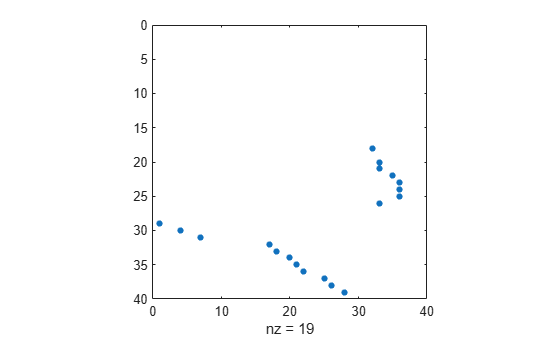Read binary image into workspace.

`BW = imread('blobs.png');`

Calculate boundaries.

`[B,L,N] = bwboundaries(BW);`

Display object boundaries in red and hole boundaries in green.

```imshow(BW); hold on; for k=1:length(B), boundary = B{k}; if(k > N) plot(boundary(:,2), boundary(:,1), 'g','LineWidth',2); else plot(boundary(:,2), boundary(:,1), 'r','LineWidth',2); end end```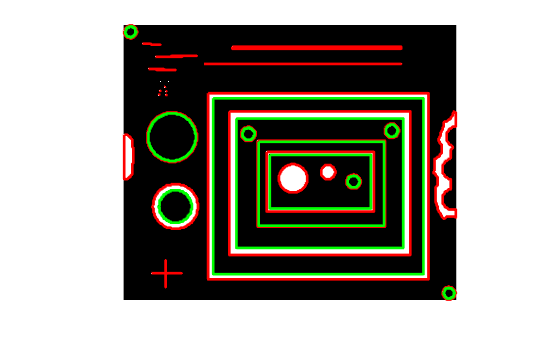Read image into workspace.

`BW = imread('blobs.png');`

Display parent boundaries in red and their holes in green.

```[B,L,N,A] = bwboundaries(BW); figure; imshow(BW); hold on; % Loop through object boundaries for k = 1:N % Boundary k is the parent of a hole if the k-th column % of the adjacency matrix A contains a non-zero element if (nnz(A(:,k)) > 0) boundary = B{k}; plot(boundary(:,2),... boundary(:,1),'r','LineWidth',2); % Loop through the children of boundary k for l = find(A(:,k))' boundary = B{l}; plot(boundary(:,2),... boundary(:,1),'g','LineWidth',2); end end end```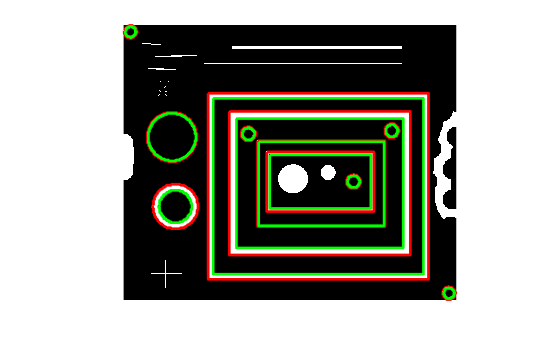## Input Arguments

collapse all

Binary input image, specified as a 2-D logical or numeric matrix. `BW` must be a binary image where nonzero pixels belong to an object and zero-valued pixels constitute the background. The following figure illustrates these components.Data Types: `single` | `double` | `int8` | `int16` | `int32` | `int64` | `uint8` | `uint16` | `uint32` | `uint64` | `logical`

Pixel connectivity, specified as one of the values in this table.

Value

Meaning

Two-Dimensional Connectivities

`4`

Pixels are connected if their edges touch. Two adjoining pixels are part of the same object if they are both on and are connected along the horizontal or vertical direction.Current pixel is shown in gray.

`8`

Pixels are connected if their edges or corners touch. Two adjoining pixels are part of the same object if they are both on and are connected along the horizontal, vertical, or diagonal direction.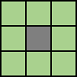Current pixel is shown in gray.

Data Types: `double`

Determine whether to search for both parent and child boundaries, specified as either of the following:

Option

Meaning

`"holes"`

Search for both object and hole boundaries. This is the default.

`"noholes"`

Search only for object (parent and child) boundaries. This can provide better performance.

Data Types: `char` | `string`

## Output Arguments

collapse all

Row and column coordinates of boundary pixels, returned as a p-by-1 cell array, where p is the number of objects and holes. The first `n` cells in `B` are object boundaries and the remaining cells are hole boundaries.

Each cell in the cell array contains a q-by-2 matrix. Each row in the matrix contains the row and column coordinates of a boundary pixel. q is the number of boundary pixels for the corresponding region.

Label matrix of contiguous regions, returned as a 2-D matrix of nonnegative integers. The kth region includes all elements in `L` that have value k. The number of objects and holes represented by `L` is equal to `max(L(:))`. The zero-valued elements of `L` make up the background.

Data Types: `double`

Number of objects found, returned as a nonnegative integer.

Data Types: `double`

Parent-child dependencies between boundaries and holes, returned as a square, sparse, logical matrix with side of length `max(L(:))`. The rows and columns of `A` correspond to the positions of boundaries stored in `B`. `A(i,j)=1` means that object `i` is a child of object `j`. The boundaries that enclose or are enclosed by the k-th boundary can be found using `A` as follows:

```enclosing_boundary = find(A(k,:)); enclosed_boundaries = find(A(:,k));```

Data Types: `double`

## Algorithms

The `bwboundaries` function implements the Moore-Neighbor tracing algorithm modified by Jacob's stopping criteria. This function is based on the `boundaries` function presented in the first edition of Digital Image Processing Using MATLAB .

 Gonzalez, R. C., R. E. Woods, and S. L. Eddins, Digital Image Processing Using MATLAB, New Jersey, Pearson Prentice Hall, 2004.